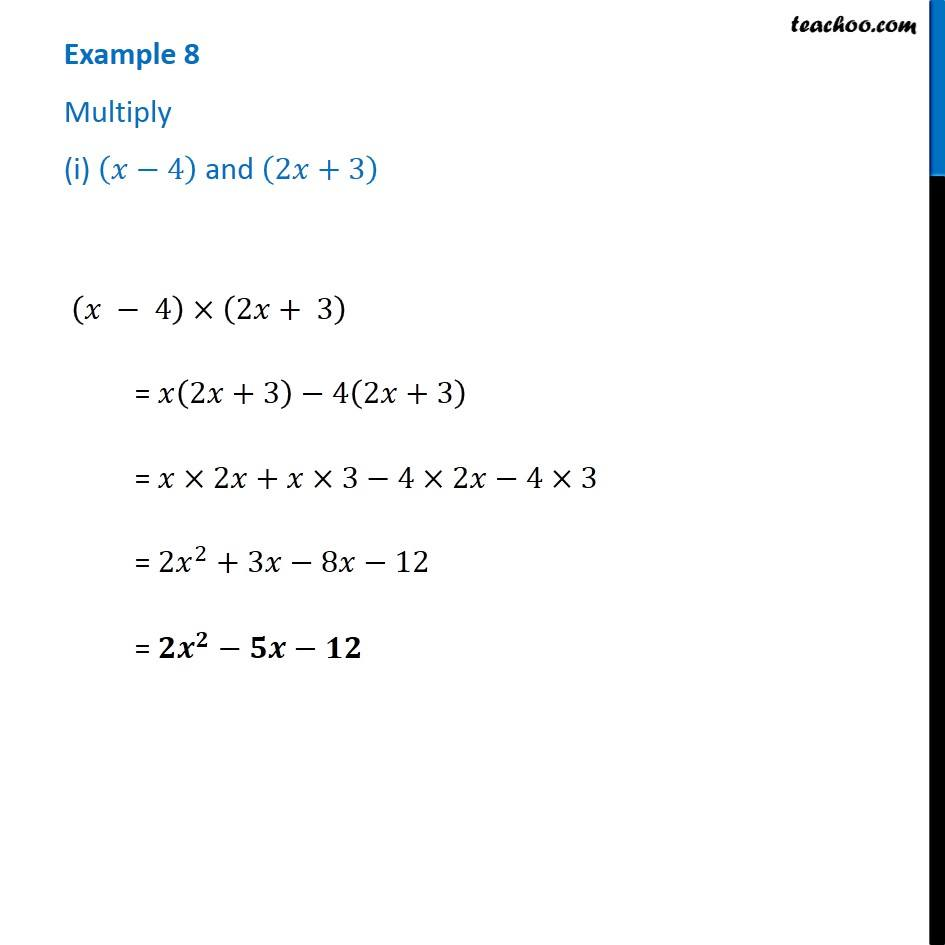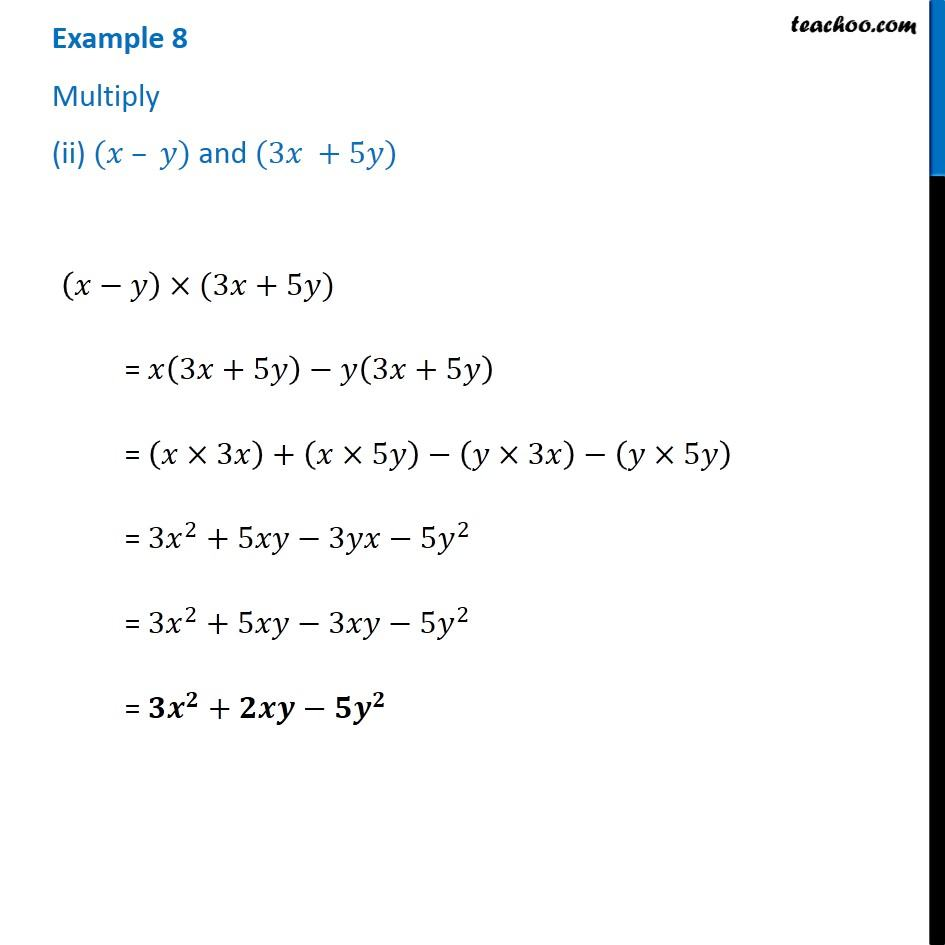1. Chapter 9 Class 8 Algebraic Expressions and Identities
2. Concept wise
3. Multiplication of Polynoimals by Polynomials

Transcript

Example 8 Multiply (i) (𝑥−4) and (2𝑥+3) (𝑥 − 4)×(2𝑥+ 3) = 𝑥(2𝑥+3)−4(2𝑥+3) = 𝑥×2𝑥+𝑥×3−4×2𝑥− 4×3 = 2𝑥^2+3𝑥−8𝑥−12 = 𝟐𝒙^𝟐−𝟓𝒙−𝟏𝟐 Example 8 Multiply (ii) (𝑥 – 𝑦) and (3𝑥 +5𝑦) (𝑥−𝑦)×(3𝑥+5𝑦) = 𝑥(3𝑥+5𝑦)−𝑦(3𝑥+5𝑦) = (𝑥×3𝑥)+(𝑥×5𝑦)−(𝑦×3𝑥)−(𝑦×5𝑦) = 3𝑥^2+5𝑥𝑦−3𝑦𝑥−5𝑦^2 = 3𝑥^2+5𝑥𝑦−3𝑥𝑦−5𝑦^2 = 𝟑𝒙^𝟐+𝟐𝒙𝒚−𝟓𝒚^𝟐

Multiplication of Polynoimals by Polynomials